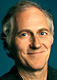Paul Saffo just sent to Farber’s IP List a pointer to “A delightful site, with great links (useful in figuring out some of the references in the text)”: What’s Special About This Number?

1 is the multiplicative identity.
2 is the only even prime.
3 s the number of spatial dimensions we live in.
4 is the smallest number of colors sufficient to color al planar maps.
5 is the number of Platonic solids.
6 is the smallest perfect number.
7 is the smallest number of faces of a regular polygon that is not constructible by straightedge and compass.
8 is the largest cube in the Fibonacci sequence.
9 is the maximum number of cubes that are needed to sum to any positive integer.

9984 is the maximum number of regions space can be divided into by 32 spheres.
9985 is the number of hyperbolic knots with 13 crossings.
9988 is the number of prime knots with 13 crossings.
9992 is the number of 2√ó2√ó2 Rubik cube positions that require exactly 5 moves to solve.
9995 has a square formed by inserting a block of digits inside itself.
9996 has a square formed by inserting a block of digits inside itself.
9998 is the smallest number n for which the concatenation of n, (n+1), … (n+21) is prime.
9999 is a Kaprekar number.

The author, Erich Friedman asks on the site for any other interesting facts about numbers. I thought immediately of the Hardy-Ramanujan number, 1729, which Wikipedia describes as follows:

1729 is known as the Hardy-Ramanujan number, after a famous anecdote of the British mathematician G. H. Hardy regarding a hospital visit to the Indian mathematician Srinivasa Ramanujan. In Hardy’s words:

“I remember once going to see him when he was ill at Putney. I had ridden in taxi cab number 1729 and remarked that the number seemed to me rather a dull one, and that I hoped it was not an unfavorable omen. “No,” he replied, “it is a very interesting number; it is the smallest number expressible as the sum of two cubes in two different ways.”

But of course, Erich already has that one :-) A really fun site for number geeks!